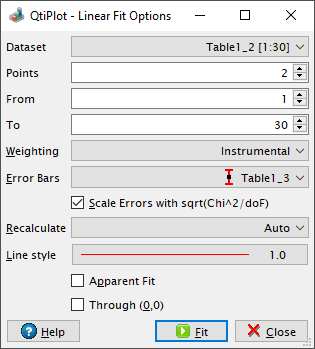Linear Fit Options

This dialog box is opened when you select the Fit Linear... command from the Analysis menu. It allows to choose the curve to fit, the number of data points for the resulting curve, the abscissa limits for the fit and the weighting method.

Figure 5-104. The Linear Fit Options dialog box.The available weighting methods are:

1. No weighting: all weighting coefficients are set to 1 (wi = 1).

2. Instrumental: the values of the associated error bars are used as weighting coefficients wi = 1/eri2, where eri are the error bar sizes stored in error bar columns. You must add error bars to the analyzed curve before performing the fit.

3. Statistical: the weighting coefficients are calculated as wi = 1/yi, where yi are the y values in the fitted data set.

4. Direct Weighting: the weighting coefficients are calculated as wi = yi, where yi are the y values in the fitted data set.

If the curve selected for the data fit operation has error bars attached, the weighting method is automatically set to Instrumental and a list box displays the names of the available error bar curves.

The Scale Errors with sqrt(Chi^2/doF) option becomes available only if the weighting method is set to something else than No weighting. This option only affects the errors on the parameters reported after the fit operation. It does not affect the fitting process or the data in any way. If checked, the reported errors on the parameters are calculated as the square root of the diagonal elements of the covariance matrix multiplied with sqrt(Chi2/(n - p)), where n is the number of data points and p the number of fit parameters. Otherwise, the reported errors equal the square root of the diagonal elements of the covariance matrix.

It is also possible to specify how the fit operation responds to modifications of the source data curve using the Recalculate list box. The available options are:

No

- No recalculation when data changes, the fit object is deleted from memory when the operation ends.

Auto

- A recalculation is automatically performed when input data changes.

Manual

- No recalculation is triggered when data changes, instead you can perform a recalculation at any time by pressing the Recalculate button from the Analysis tab of the plot details dialog.

The recalculation mode can be changed later on via the Analysis tab of the plot details dialog.

If the Apparent Fit option is checked, QtiPlot uses the apparent values for fitting, according to the current axis scales. For example, select this box to fit exponentially decaying data with a straight line fit when data are plotted on a log scale. When this check box is selected and the data has error values associated with it, QtiPlot uses the larger of the positive/negative errors as weight. Apparent Fit is only useful when you fit from a graph and change the plot axis type (from Linear to Log10, for example). If you check this option, QtiPlot will first transform raw data into new data space as specified in the graph axis type, and then fit the curve with the new data. Otherwise, QtiPlot always fits raw data directly, regardless of the axis type. Apparent fit is equivalent to direct fit if you first transform raw data on the worksheet, and leads to completely different results from direct fit if your graph axis is non-linear.

If the option Through (0,0) is checked, QtiPlot uses the linear slope function y = A*x in order to perform the fit, instead of the default equation (y = A*x + B). This is equivalent to forcing the fit line to pass through the point with coordinates (0,0).

The numerical result of the fit operation will be displayed in the Log Panel.